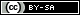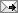## Gavin R. Putland,  BE PhD

 Thursday, May 07, 2020 (Comment)

# Exact derivation of Kirchhoff's integral theorem and diffraction formula using high-school math

#### Abstract (Version 4; April 9, 2020)

A theory of diffraction is developed from three premises: causality (including the assumption that the wave function had a beginning), superposition, and the form of the wave function due to a monopole source. It is shown that the wave function in a region R, due to sources outside R (that is, in R′), is identical to that due to a distribution of spatiotemporal dipole (STD) sources on the surface S separating R′ and R, facing R, with a strength density given by the original wave function on S (divided by 4π, under the adopted definition of strength). The Kirchhoff integral theorem and consequent diffraction formulae are obtained by superposing the elemental wave functions due to the sources on S. Whereas D.A.B. Miller (1991) justified the STDs by comparison with Kirchhoff's integral, I derive the integral (with a near-primary-source correction to Miller's form) solely from the STDs. Diffraction by an aperture in an opaque screen is modeled by assuming that we retain only the sources on that part of S which spans the aperture. This assumption avoids the notorious inconsistency in Kirchhoff's boundary conditions (1882), but gives the same diffraction integral. It also yields the consistent saltus conditions of Kottler (1923) and a more direct saltus description of the aperture, giving the same solution in R. Finally, the diffraction integral for a monopole primary source is transformed to a geometrical-optics term plus an integral along the edge of the aperture, and applied to the Poisson-Arago spot. The transformation (due to Maggi and Rubinowicz) is first derived from a far-field/short-wavelength assumption, but later re-derived exactly for general time-dependence, and shown to be a valid approximation for a weakly directional primary source.

Full text: doi.org/10.5281/zenodo.3659325.TweetReturn to Contents